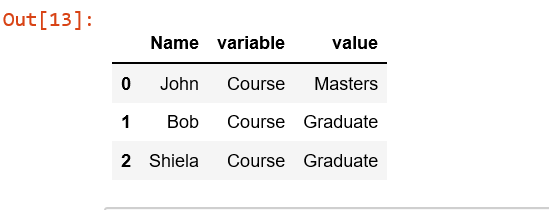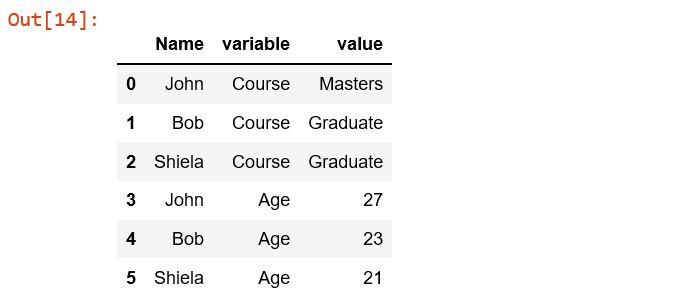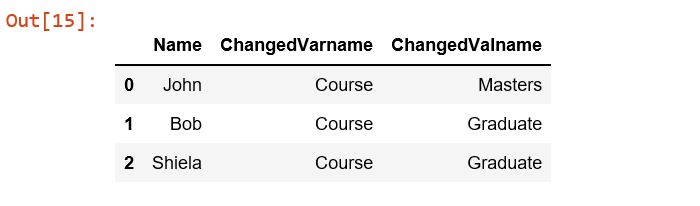# Python | Pandas.melt()

To make analysis of data in table easier, we can reshape the data into a more computer-friendly form using Pandas in Python. Pandas.melt() is one of the function to do so..
Pandas.melt() unpivots a DataFrame from wide format to long format.
melt() function is useful to massage a DataFrame into a format where one or more columns are identifier variables, while all other columns, considered measured variables, are unpivoted to the row axis, leaving just two non-identifier columns, variable and value.
Syntax :

```pandas.melt(frame, id_vars=None, value_vars=None,
var_name=None, value_name='value', col_level=None)```

Parameters:

frame : DataFrame
id_vars[tuple, list, or ndarray, optional] : Column(s) to use as identifier variables.
value_vars[tuple, list, or ndarray, optional]: Column(s) to unpivot. If not specified, uses all columns that are not set as id_vars.
var_name[scalar]: Name to use for the ‘variable’ column. If None it uses frame.columns.name or ‘variable’.
value_name[scalar, default ‘value’]: Name to use for the ‘value’ column.
col_level[int or string, optional]: If columns are a MultiIndex then use this level to melt.

Example:

 `# Create a simple dataframe ` ` `  `# importing pandas as pd ` `import` `pandas as pd ` ` `  `# creating a dataframe ` `df ``=` `pd.DataFrame({``'Name'``: {``0``: ``'John'``, ``1``: ``'Bob'``, ``2``: ``'Shiela'``}, ` `                   ``'Course'``: {``0``: ``'Masters'``, ``1``: ``'Graduate'``, ``2``: ``'Graduate'``}, ` `                   ``'Age'``: {``0``: ``27``, ``1``: ``23``, ``2``: ``21``}}) ` `df ``# Name is id_vars and Course is value_vars ` `pd.melt(df, id_vars ``=``[``'Name'``], value_vars ``=``[``'Course'``]) ``# multiple unpivot columns ` `pd.melt(df, id_vars ``=``[``'Name'``], value_vars ``=``[``'Course'``, ``'Age'``]) ``# Names of ‘variable’ and ‘value’ columns can be customized ` `pd.melt(df, id_vars ``=``[``'Name'``], value_vars ``=``[``'Course'``], ` `              ``var_name ``=``'ChangedVarname'``, value_name ``=``'ChangedValname'``) `Attention geek! Strengthen your foundations with the Python Programming Foundation Course and learn the basics.

To begin with, your interview preparations Enhance your Data Structures concepts with the Python DS Course.

My Personal Notes arrow_drop_upCheck out this Author's contributed articles.

If you like GeeksforGeeks and would like to contribute, you can also write an article using contribute.geeksforgeeks.org or mail your article to contribute@geeksforgeeks.org. See your article appearing on the GeeksforGeeks main page and help other Geeks.

Please Improve this article if you find anything incorrect by clicking on the "Improve Article" button below.

Article Tags :

3

Please write to us at contribute@geeksforgeeks.org to report any issue with the above content.